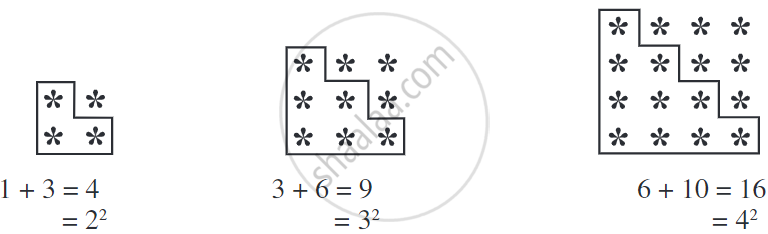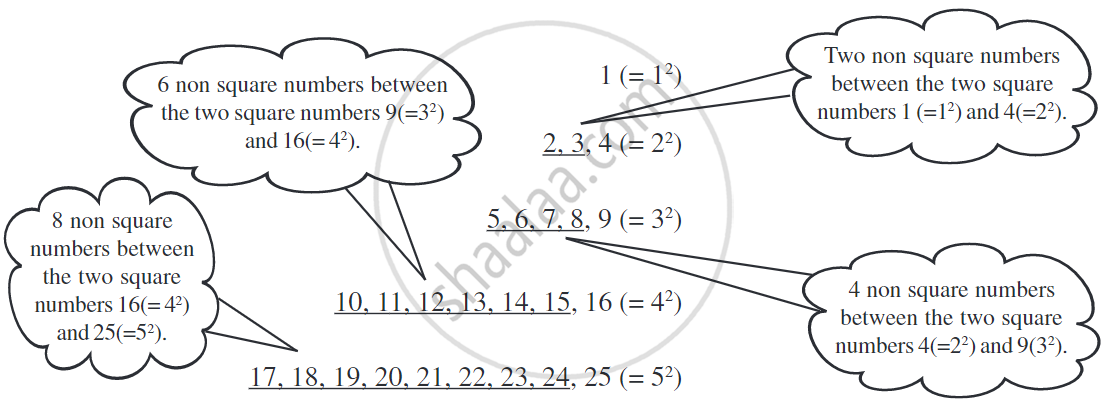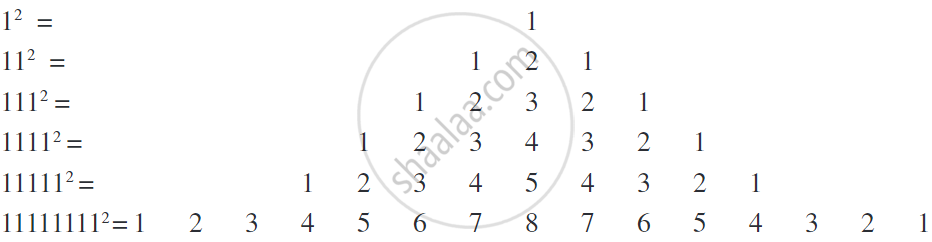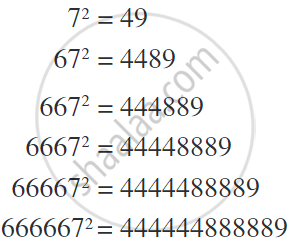# Some More Interesting Patterns of Square Number

## Notes

### Some More Interesting Patterns of Square Number:

If we combine two consecutive triangular numbers, we get a square number, like#### 2. Numbers between square numbers:Between 12(=1) and 22(= 4), there are two (i.e., 2 × 1) non-square numbers 2, 3.
Between 22(= 4) and 32(= 9) there are four (i.e., 2 × 2) non-square numbers 5, 6, 7, 8.
Now, 32 = 9, 42 = 16.
Therefore, 42 – 32 = 16 – 9 = 7.
Between 9(= 32) and 16(= 42), the numbers are 10, 11, 12, 13, 14, 15 that is, six non-square numbers which is 1 less than the difference of two squares.
We have 42 = 16 and 52 = 25.
Therefore, 52 – 42 = 9.
Between 16 (= 42) and 25(= 52), the numbers are 17, 18,..., 24 that is, eight non-square numbers which is 1 less than the difference of two squares.

If we think of any natural number n and (n + 1), then,
(n + 1)2 – n2 = (n2 + 2n + 1) – n2 = 2n + 1.
We find that between n2 and (n + 1)2  there are 2n numbers which is 1 less than the difference of two squares.
Thus, in general, we can say that there are 2n non-perfect square numbers between the squares of the numbers n and (n + 1), where n is any natural number.

The sum of first n odd natural numbers is n2.

1 [one odd number] = 1 = 12

1 + 3 [sum of first two odd numbers] = 4 = 22

1 + 3 + 5 [sum of first three odd numbers] = 9 = 32

If a natural number cannot be expressed as a sum of successive odd natural numbers starting with 1, then it is not a perfect square.

25 = 1 + 3 + 5 + 7 + 9. Also, 25 is a perfect square.

#### 4. A sum of consecutive natural numbers:

We can express the square of any odd number as the sum of two consecutive positive integers (n^2 – 1)/2 and (n^2 + 1)/2.

For Example,

112 = 121 = 60 + 61 = (11^2 – 1)/2 + (11^2 + 1)/2.

152 = 225 = 112 + 113 = (15^2 – 1)/2 + (15^2 + 1)/2.

#### 5. Product of two consecutive even or odd natural numbers:

Product of two consecutive even or odd natural numbers are calculated as (a + 1) × (a – 1) = a2 – 1 where a is a natural number and (a + 1), (a – 1) are two consecutive even or odd natural numbers.

For example,

29 × 31 = (30 – 1) × (30 + 1) = 302 – 1.

44 × 46 = (45 – 1) × (45 + 1) = 452 – 1.

#### 6. Some more patterns in square numbers:

Observe the squares of numbers; 1, 11, 111,... etc. They give a beautiful pattern:Another interesting pattern.If you would like to contribute notes or other learning material, please submit them using the button below.

### Shaalaa.com

Pattern of Square Numbers - Part 1 [00:07:09]
S
0%